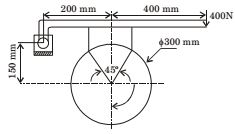## Machine Design Miscellaneous

#### Machine Design

1. A thin spherical pressure vessel of 200 mm diameter and 1 mm thickness is subjected to an internal pressure varying from 4 to 8 MPa. Assume that the yield, ultimate, and endurance strength of material are 600,800 and 400 MPa respectively. The factor of safety as per Goodman's relation is

1. Stress induced,

 σ1 = σ2 = pr 2t

 σ1max = 8 × 100 = 400MPa 2 × 1

 σ1min = 4 × 100 = 200MPa 2 × 1

σ2max = 400 MPa
σ2min = 200 MPa
σ1m = 300 MPa
σ1a = 100 MPa
σ2m = 300 MPa
σ2a = 100 MPa
Equivalent stress are as follows:
σme = √σ1m² + σ2m² − σ1mσ2m
= σme = √300² + 300² − 300 × 300
= 300 Mpa
Similarly, σae = 100 MPa
From Goodman equation,
 σae + σme = 1 Se Sut n

 ⇒ 100 + 300 = 1 400 800 n

= n = 1.6

##### Correct Option: B

Stress induced,

 σ1 = σ2 = pr 2t

 σ1max = 8 × 100 = 400MPa 2 × 1

 σ1min = 4 × 100 = 200MPa 2 × 1

σ2max = 400 MPa
σ2min = 200 MPa
σ1m = 300 MPa
σ1a = 100 MPa
σ2m = 300 MPa
σ2a = 100 MPa
Equivalent stress are as follows:
σme = √σ1m² + σ2m² − σ1mσ2m
= σme = √300² + 300² − 300 × 300
= 300 Mpa
Similarly, σae = 100 MPa
From Goodman equation,
 σae + σme = 1 Se Sut n

 ⇒ 100 + 300 = 1 400 800 n

= n = 1.6

1. A carpenter glues a pair of cylindrical wooden logs by bonding their end faces at an angle of θ = 30° as shown in the figure.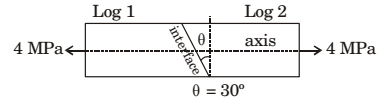The glue used at the interface fails if
Criterion 1: the maximum normal stress exceeds 2.5 MPa
Criterion 2: the maximum shear stress exceeds 1.5 MPa
Assume that the interface fails before the logs fail. When a uniform tensile stress of 4 MPa is applied, the interface

1. Normal stress on inclined plane
σ = σx cos² θ
= 4 × cos²30 = 3 MPa

 Shear stress on inclined plane τ = σx sin2θ 2

= 2 × sin 60° = 1.73 MPa
Since both the stress exceeds the given limits, answer is option (c).

##### Correct Option: C

Normal stress on inclined plane
σ = σx cos² θ
= 4 × cos²30 = 3 MPa

 Shear stress on inclined plane τ = σx sin2θ 2

= 2 × sin 60° = 1.73 MPa
Since both the stress exceeds the given limits, answer is option (c).

1. If σ1 and σ3 are the algebraically largest and smallest principal stresses respectively, the value of the maximum shear stress is

1. Maximum shear stress = Radius of Mohr’s Circle (for plane stress condition)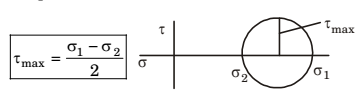##### Correct Option: B

Maximum shear stress = Radius of Mohr’s Circle (for plane stress condition)1. A pin jointed uniform rigid rod of weight W and length L is supported horizontally by an external force F as shown in the figure below. The force F is suddenly removed. At the instant of force removal, the magnitude of vertical reaction developed at the support is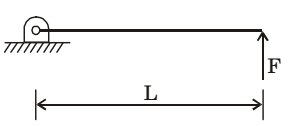1.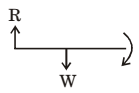I = 1 W L2 ; W × L Iα  ⇒  α = 3g 3 g 2 2L

 ∴  Linear acceleration at centre = α × L = 3g 2 4

 ∴ Inertial force at centre = 3 W 4

 ∴  Reaction at support = W – 3W = W 4 4

##### Correct Option: BI = 1 W L2 ; W × L Iα  ⇒  α = 3g 3 g 2 2L

 ∴  Linear acceleration at centre = α × L = 3g 2 4

 ∴ Inertial force at centre = 3 W 4

 ∴  Reaction at support = W – 3W = W 4 4

1. A block-brake shown below has a face width of 300 mm and a mean coefficient of friction of 0.25. For an activating force of 400 N, the braking torque in Nm is